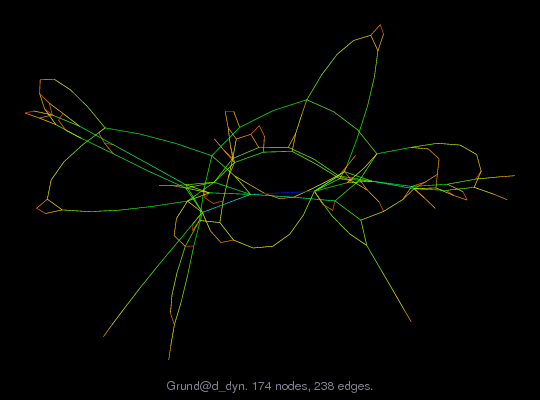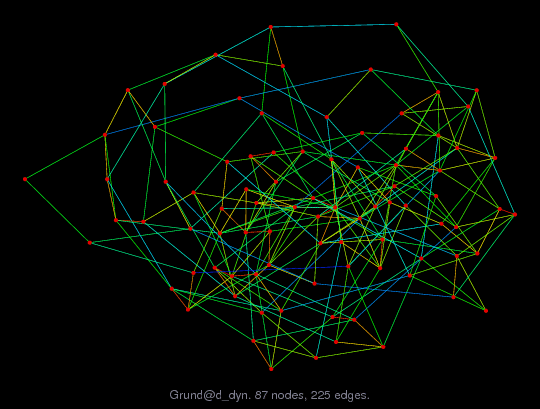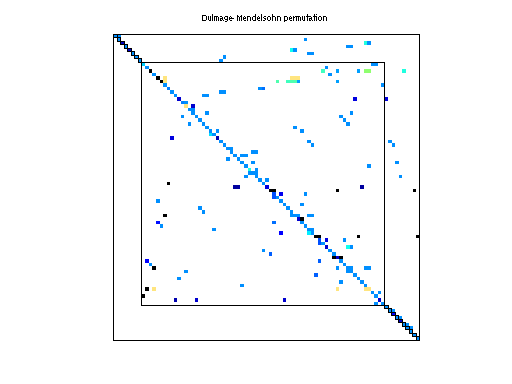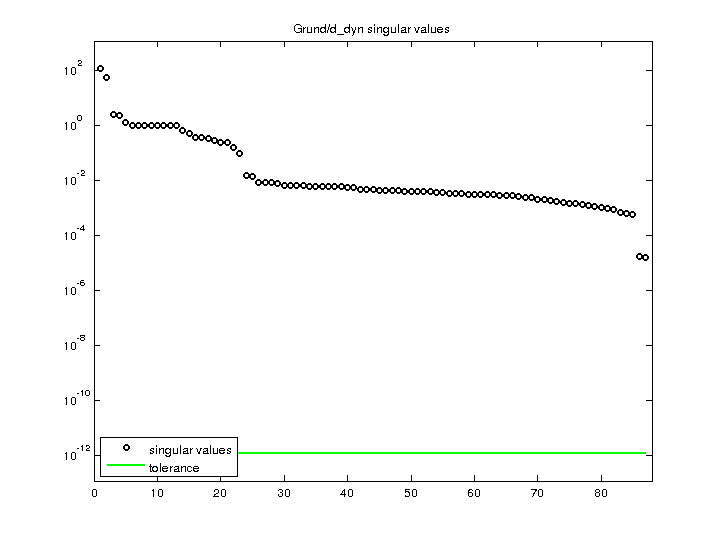Matrix: Grund/d_dyn

Description: Unsymmetric Matrix d_dyn, F. Grund, Dec 1994.(bipartite graph drawing) (graph drawing of A+A')• Matrix group: Grund
• download as a MATLAB mat-file, file size: 2 KB. Use UFget(462) or UFget('Grund/d_dyn') in MATLAB.

 Matrix properties number of rows 87 number of columns 87 nonzeros 230 structural full rank? yes structural rank 87 # of blocks from dmperm 19 # strongly connected comp. 1 explicit zero entries 8 nonzero pattern symmetry 8% numeric value symmetry 2% type real structure unsymmetric Cholesky candidate? no positive definite? no

 author F. Grund editor F. Grund date 1997 kind chemical process simulation problem 2D/3D problem? no

 Additional fields size and type b full 87-by-1

 Ordering statistics: result nnz(chol(P*(A+A'+s*I)*P')) with AMD 917 Cholesky flop count 1.4e+04 nnz(L+U), no partial pivoting, with AMD 1,747 nnz(V) for QR, upper bound nnz(L) for LU, with COLAMD 239 nnz(R) for QR, upper bound nnz(U) for LU, with COLAMD 431

Note that all matrix statistics (except nonzero pattern symmetry) exclude the 8 explicit zero entries.

 SVD-based statistics: norm(A) 112.905 min(svd(A)) 1.52251e-05 cond(A) 7.41575e+06 rank(A) 87 sprank(A)-rank(A) 0 null space dimension 0 full numerical rank? yes

 singular values (MAT file): click here SVD method used: s = svd (full (A)) ; status: ok